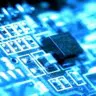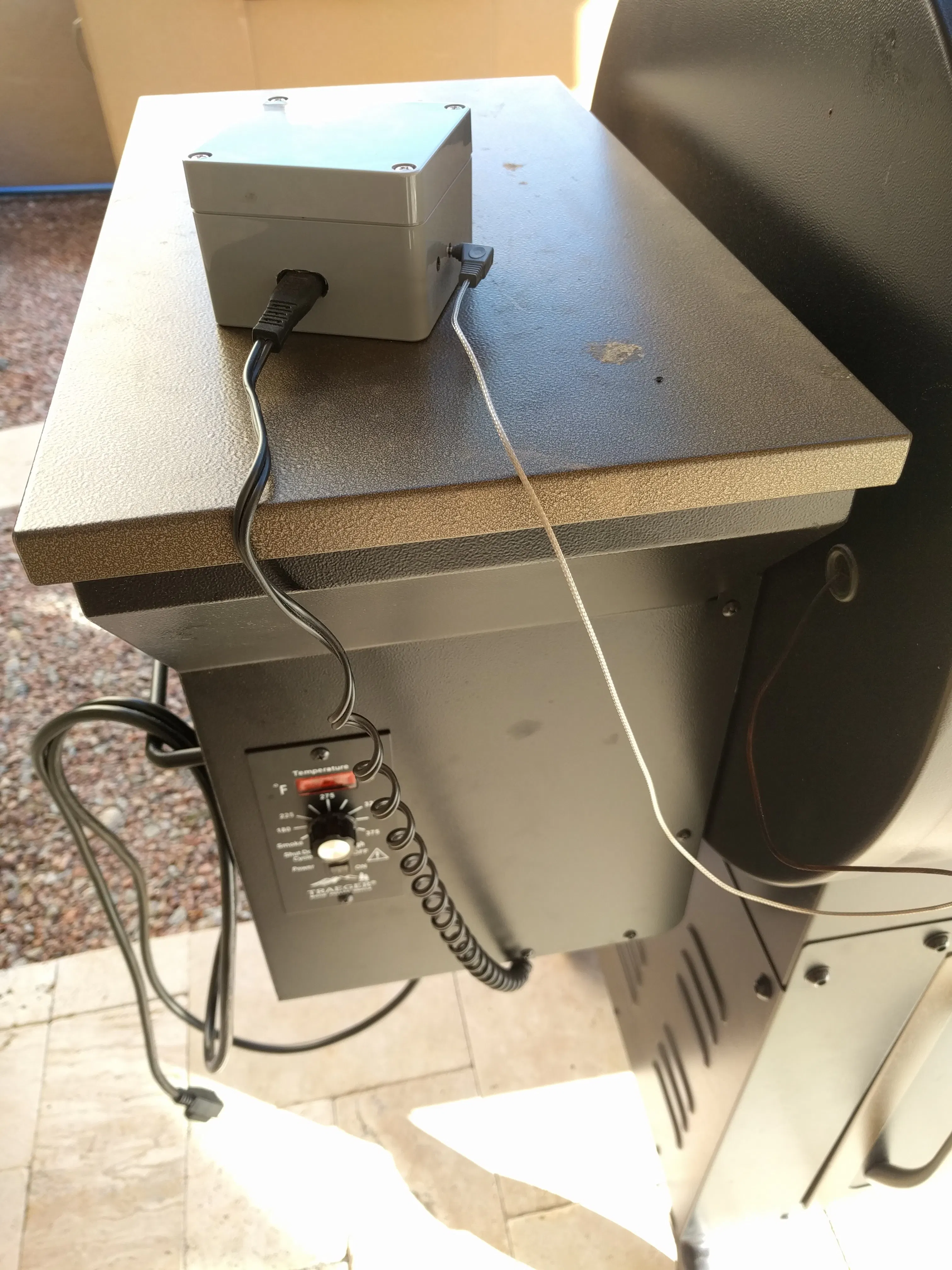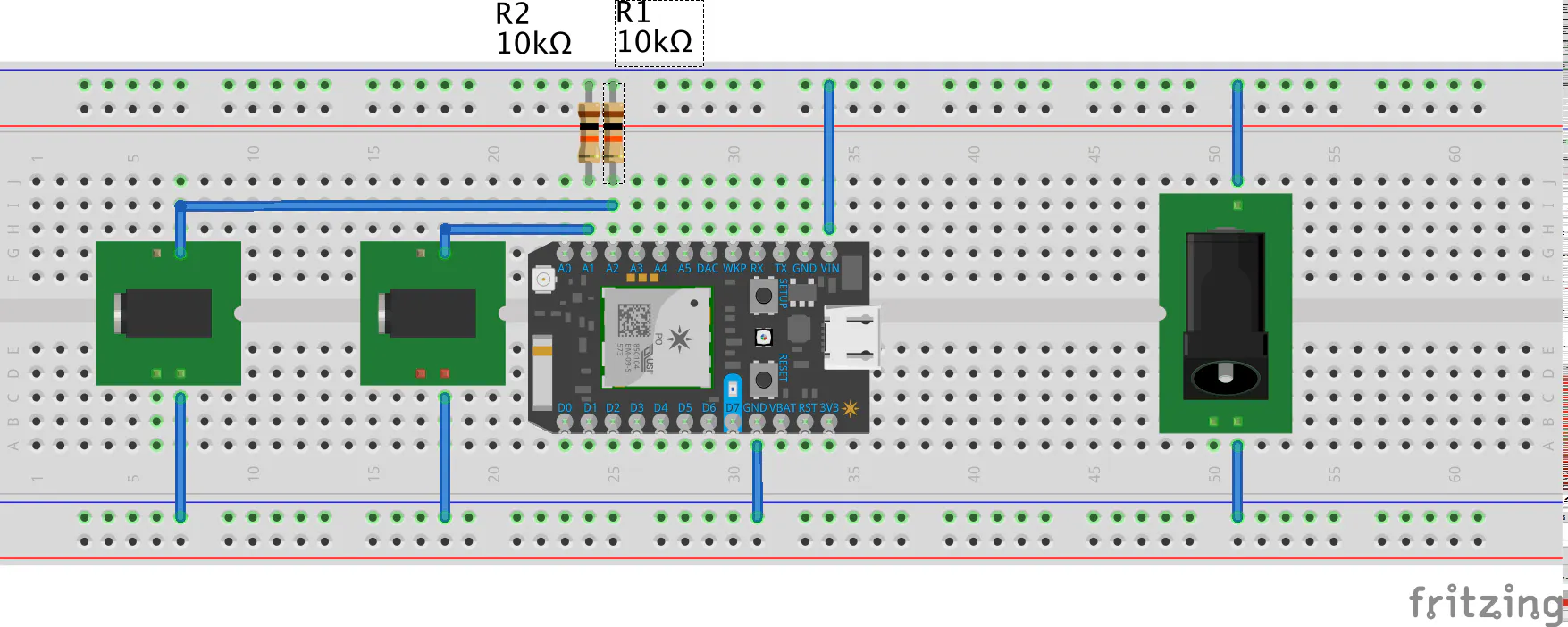# BBQ Monitor

IntermediateShowcase (no instructions)3 hours2,818## Things used in this project

### Hardware componentsParticle Photon
×1
 Temperature probe
×1
 2.5mm audio jack.
×1

### Hand tools and fabrication machinesSoldering iron (generic)

## Schematics

### BBQ schematic## Code

### Photon firmware

C/C++
Comile and upload via the web IDE for Photon devices.
```// these values are for Maverick PR-003 probe, which works with devices Et-71/72/73/901
//float steinhart_a = 0.00024723753;
//float steinhart_b = 0.00023402251;
//float steinhart_c = 0.00000013879768;

//float c;
//float k;
//float f;
int boardLed = D7;
int therm = A0;
int therm2 = A1;
int resistance;
int resistance2;

void setup() {
pinMode(boardLed,OUTPUT);
pinMode(therm,INPUT);
pinMode(therm2,INPUT);
//Particle.variable("therm", &measure, INT);
Particle.variable("resistance", &resistance, INT);
Particle.variable("resistance2", &resistance2, INT);
RGB.control(true);
pinMode(D0, INPUT);
}

void loop() {
int measure = 0;
for (int x=0; x<8; x++) {
delay(10);
}
measure /= 8;
//resistance = 150000;
resistance = int(10000.0 / (4095.0/measure - 1.0));

int measure2 = 0;
for (int x=0; x<8; x++) {
delay(10);
}
measure2 /= 8;
//resistance = 150000;
resistance2 = int(10000.0 / (4095.0/measure2 - 1.0));

// Steinhart equations for calculating temp.
//float ln_resistance = ln(resistance);
//k = 1/(steinhart_a+steinhart_b*ln_resistance+steinhart_c*(ln_resistance*ln_resistance*ln_resistance));
//c = k-273.15;
//f = c*1.8+32;
//char f_char;
char m_char;
char r_char, r_char2;
//sprintf(f_char,"%d", f);
//sprintf(m_char,"%d", measure);
sprintf(r_char,"%d", resistance);
sprintf(r_char2,"%d", resistance2);
//Particle.publish("therm",m_char);
Particle.publish("resistance",r_char);
Particle.publish("resistance2",r_char2);
//Particle.publish("temp",f_char);
digitalWrite(boardLed,HIGH);
delay(500);
digitalWrite(boardLed,LOW);
delay(10000);
}
}
```